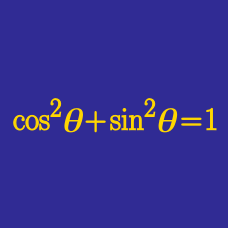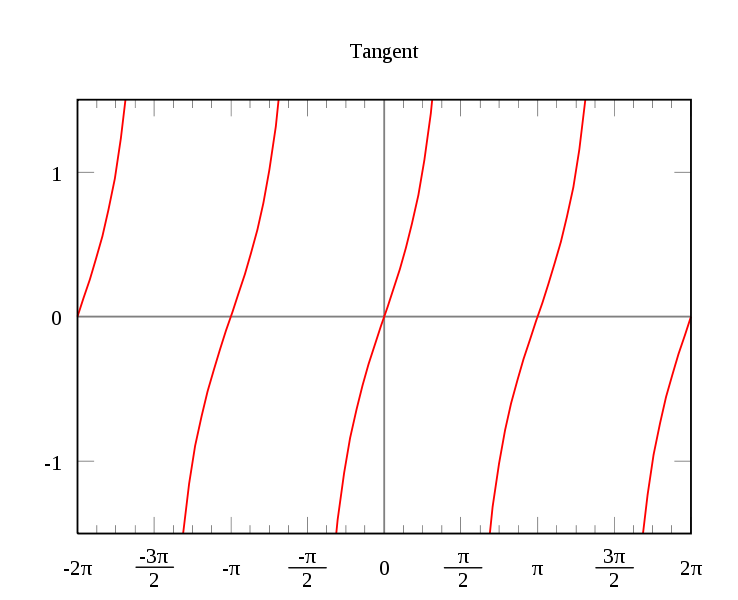Geometry

# Fundamental Trigonometric Identities: Level 2 Challenges

Find the value of

$\cos^2 45^{\circ} +\sin^2 45^{\circ}.$Simplify $\tan\left(A + \frac{\pi}{2}\right).$

If $\sin A +\sin^{2}A=1,$ find the value of $\cos ^{2} A + \cos ^{4} A.$

$\ln(\tan1^{\circ})+ \ln(\tan2^{\circ})+\ldots+\ln(\tan88^{\circ})+\ln(\tan89^{\circ})= \ ?$

What is the value of $\sin 20^{\circ}(\tan 10^{\circ}+\cot 10^{\circ})$?

×

Problem Loading...

Note Loading...

Set Loading...